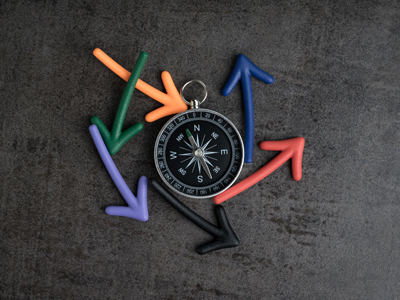Which direction does the straight line starting at (-5, 2) and ending at (-2, -1) point in?

# Position 3 (Difficult)

This is the third quiz in our Difficult series of Eleven Plus maths quizzes on Position. If you have already played the first two and our Very Easy, Easy, and Medium ones, then this one shouldn’t be too hard. Just remember what you have learned about directions, points on a compass and coordinates, and you will be fine!

Before you start, here’s a tip: get hold of some graph paper, a ruler and a pencil. There will be some questions in this quiz which will be much easier if you mark out the given coordinate points, or draw the shapes required. Some of us can visualise these in our heads, but for mere mortals like me, pencils and paper make the world of difference!

As ever, make sure you read each question carefully before you choose your answer, and take your time – there are no extra marks for finishing quickly!

Good luck!

1.
A circle with centre (-7, 2) and diameter 4 cm is drawn on the coordinate plane. Which points will the circle pass through?
(-3, 2), (-7, 6), (-11, 2), (-7, -2)
(5, -2), (7, -4), (9, -2), (7, 0)
(3, -2), (7, -6), (11, -2), (7, 0)
(-5, 2), (-7, 4), (-9, 2), (-7, 0)
The radius is half the diameter = 2 cm; therefore, the circle will pass through those points that are at a distance of 2 cm from its centre (-7, 2)
2.
Which direction does the straight line starting at (-5, 2) and ending at (-2, -1) point in?
SW
SE
NW
NE
A diagram will help a lot in doing these questions!
3.
What are the coordinates of the point that is the same distance away from the x-axis as the point (0, -8)?
(0, 8)
(8, 8)
(8, 0)
(-8, 0)
(0, -8) is at 8 on the negative y-axis: 8 units below the x-axis. (0, 8) is at 8 on the positive y-axis: 8 above the x-axis
4.
How many degrees are there in seven-half rotations?
315°
630°
1,260°
2,520°
There are 180° in a half rotation: 180 x 7 = 1,260°
5.
If you join up the points A (-6, -3), B (-6, -5), C (-4, -5), D (-4, -3) with straight lines you will get a square that lies in a certain quadrant. If the square is rotated anticlockwise through 90° about the point (0, 0), which quadrant will it end up in and what will its new coordinates be?
A (3, 6) B (5, 6), C (5, 4) D (3, 4) in the first quadrant
A (-3, 6), B (-5, 6), C (-5, 4) D (-3, 4) in the second quadrant
A (3, -6), B (5, -6), C (5, -4) D (3, -4) in the third quadrant
A (3, -6), B (5, -6), C (5, -4) D (3, -4) in the fourth quadrant
The square is initially in the third quadrant. Join up the four corners with straight lines (the lengths of which should be 2 units). to the centre of rotation (0, 0). Now rotate your paper 90° anticlockwise and watch where their end points end up: these will give you the positions of the new coordinates of the square. Remember to relabel your axes!
6.
Which coordinates are SW of (3, 12)?
(-1, -10)
(1, -10)
(-1, 10)
(1, 10)
Starting at (3, 12) draw a line 225° from North (45 x 5 = 215). All points along this line (including 1, 10) lie SW of (3, 12)
7.
You are facing SW. If you turn through 225° anticlockwise and then 90° clockwise, which direction will you be facing now?
E
NE
SE
N
'Anticlockwise' means that you turn (rotate) in the opposite direction to which the hands of the clock turn: the hands of the clock turn 'clockwise'.
225° = five lots of 45° (five-eighths of a turn) anticlockwise makes you face N. 90° clockwise = one-quarter of a turn, makes you face E
8.
Cassie is drawing a straight line path starting at point A (-1, -1). She then moves as follows: 2 cm West to point B, SE to point C which is due South of point A, and 8 cm South to point D. What are the coordinates of point D?
(-11, -1)
(1, -11)
(-1, -11)
(-1, 11)
The best way to work this out is with a diagram – it’s very hard to keep all that information in your head!
9.
Which of the following is the angle between E and NW?
115°
125°
135°
145°
Three lots of 45° anticlockwise. If you rotated in the clockwise direction, it would be five lots of 45° = 225°. Whenever you can, always give the smallest angle. Note: 225° anticlockwise + 135° clockwise = 360°
10.
You are facing SE. If you turn through 45° anticlockwise and then 315° clockwise, which direction will you be facing now?
N
NE
E
SE
45° anticlockwise makes you face E. 315° clockwise = 7 lots of 45° = (seven-eighths of a turn) makes you face NE
Author:  Frank Evans